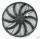# Day

What part of the day is 23 hours 22 minutes? Express as a decimal number.

Result

x =  0.97

#### Solution:

$x=(23+22/60)/24 = 0.97$

Our examples were largely sent or created by pupils and students themselves. Therefore, we would be pleased if you could send us any errors you found, spelling mistakes, or rephasing the example. Thank you!

Leave us a comment of this math problem and its solution (i.e. if it is still somewhat unclear...):Be the first to comment!#### Following knowledge from mathematics are needed to solve this word math problem:

Need help calculate sum, simplify or multiply fractions? Try our fraction calculator.

## Next similar math problems:

1. In fractionsAn ant climbs 2/5 of the pole on the first hour and climbs 1/4 of the pole on the next hour. What part of the pole does the ant climb in two hours?
2. FractionDetermine for what x fraction ?:
3. WithdrawalIf I withdrew 2/5 of my total savings and spent 7/10 of that amount. What fraction do I have in left in my savings?
4. Fraction to decimalWrite the fraction 3/22 as a decimal.
5. Fraction and a decimalWrite as a fraction and a decimal. One and two plus three and five hundredths
6. Pizza 4Marcus ate half pizza on monday night. He than ate one third of the remaining pizza on Tuesday. Which of the following expressions show how much pizza marcus ate in total?
7. To improper fractionChange mixed number to improper fraction a) 1 2/15 b) -2 15/17
8. Mixed2improperWrite the mixed number as an improper fraction. 166 2/3
9. FanThe fan has a speed of 210 RPM. Calculate for time of one fan period.
10. Reduce to lowest termsReduce to lowest terms : 32/124
11. Write 2Write 791 thousandths as fraction in expanded form.
12. Product of two fractionsProduct of two fractions is 9 3/5 . If one of the fraction is 9 3/7. Find the other fraction.
13. Passenger boatTwo-fifths of the passengers in the passenger boat were boys. 1/3 of them were girls and the rest were adult. If there were 60 passengers in the boat, how many more boys than adult were there?
14. Cupcakes 2Susi has 25 cupcakes. She gives 4/5. How much does she have left?
15. TeacherTeacher Rem bought 360 pieces of cupcakes for the outreach program of their school. 5/9 of the cupcakes were chocolate flavor and 1/4 wete pandan flavor and the rest were a vanilla flavor. How much more pandan flavor cupcakes than vanilla flavor?
16. Fractions 4How many 2/3s are in 6?
17. Pizza 5You have 2/4 of a pizza and you want to share it equally between 2 people how much pizza does each person get?